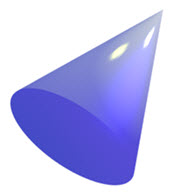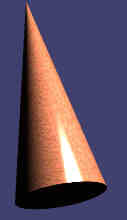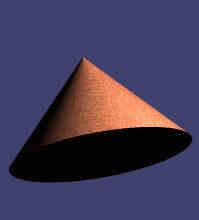# Cone

Go to Surface Area or Volume.

### Cone Facts

Notice these interesting things:

• It has a circle at one end
• And a point at the other end
• And a curved side
• It is not a polyhedron as it has a curved surfaceimages/poly-gl.js?mode=cone• The pointy end of a cone is called the apex
• The flat part is the base

An object shaped like a cone is said to be conical

images/cone-sweep.js

## A Cone is a Rotated Triangle

A cone can be made by rotating a triangle!

The triangle is a right-angled triangle, and it gets rotated around one of its two short sides.

The side it rotates around is the axis of the cone.

## Right vs Oblique Cone

When the apex is aligned on the center of the base it is a Right Cone otherwise it is an Oblique Cone:## Surface Area of a ConeThe Surface Area has two parts:

• The Base Area = π × r2
• The Side Area = π × r × s

Which together makes:

Surface Area = π × r × (r + s)

Note: we can calculate s = √(r2+h2)

### Example: h = 7 and r = 2

Surface Area of Base= π × r2
= π × 22
= 4π
12.57

Surface Area of Side= π × r × √(r2+h2)
= π × 2 × √(22+72)
= π × 2 × √(4+49)
= 2π√(53)
45.74

Total Surface Area ≈ 12.57 + 45.74 ≈ 58.31

## Volume of a Cone

Volume = 1 3 π × r2 × h

### Example: h = 7 and r = 2

Volume= 1 3 π × r2 × h
= 1 3 π × 22 × 7
= 28 3 π
29.32

Play with it here. The formula also works when it "leans over" (oblique) but remember that the height is always at right angles to the base:

## Volume of a Cone vs CylinderThe volume formulas for cones and cylinders are very similar:

 The volume of a cylinder is: π × r2 × h The volume of a cone is: 1 3 π × r2 × h

So a cone's volume is exactly one third ( 1 3 ) of a cylinder's volume.

You should order your ice creams in cylinders, not cones, you get 3 times as much!

## Like a Pyramid

A cone is also like a pyramid with an infinite number of sides, see Pyramid vs Cone.

## Different Shaped Cones## Construction ConeThis is almost a cone, but the top is chopped off (called a "truncated cone").

Also it has a wider base added so it doesn't fall over!

873,874,875,876, 1833, 1834, 3399, 3400, 3401, 3402